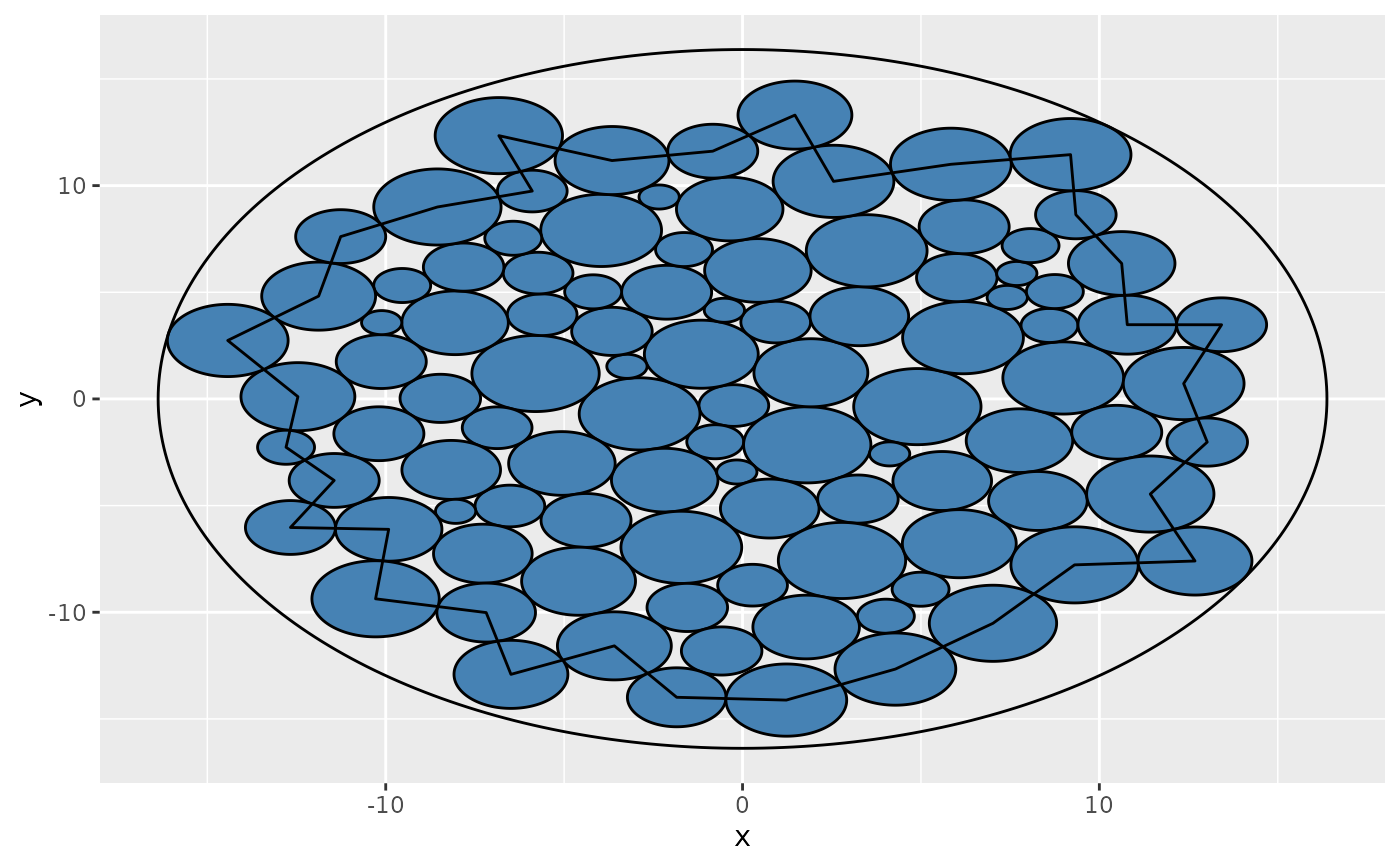This function is a direct interface to the circle packing algorithm used by layout_tbl_graph_circlepack. It takes a vector of sizes and returns the x and y position of each circle as a two-column matrix.

pack_circles(areas)

## Arguments

areas

A vector of circle areas

## Value

A matrix with two columns and the same number of rows as the length of the "areas" vector. The matrix has the following attributes added: "enclosing_radius" giving the radius of the smallest enclosing circle, and "front_chain" giving the terminating members of the front chain (see Wang et al. 2006).

## Examples

library(ggforce)
sizes <- sample(10, 100, TRUE)

position <- pack_circles(sizes)
data <- data.frame(x = position[,1], y = position[,2], r = sqrt(sizes/pi))

ggplot() +
geom_circle(aes(x0 = x, y0 = y, r = r), data = data, fill = 'steelblue') +
geom_circle(aes(x0 = 0, y0 = 0, r = attr(position, 'enclosing_radius'))) +
geom_polygon(aes(x = x, y = y),
data = data[attr(position, 'front_chain'), ],
fill = NA,
colour = 'black')